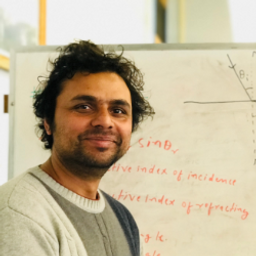Ask your homework questions to teachers and professors, meet other students, and be entered to win \$600 or an Xbox Series X 🎉Join our Discord!University of Wisconsin - Milwaukee

# The near point of a person’s eye is 60.0 cm. To see objects clearly at a distance of 25.0 cm, what should be the (a) focal length and (b) power of the appropriate corrective lens? (Neglect the distance from the lens to the eye.)

## \begin{array}{l}{f=42.9 \mathrm{cm}} \\ {D=+2.33 \text { diopter } s}\end{array}

#### Topics

Electromagnetic Waves

Wave Optics

### Discussion

You must be signed in to discuss.
##### Top Physics 103 Educators##### Andy C.

University of Michigan - Ann Arbor##### Marshall S.

University of Washington##### Aspen F.

University of Sheffield##### Jared E.

University of Winnipeg

### Video Transcript

we have a near point off a person, which is 60 centimeter. And if the person wants to be able to see an object from a distance of 25 centimetre, he's gonna have to use a corrective. Lance. So first of all, 25 centimeter is gonna be the object of sense, right? 25 centimeter. And if you he uses the corrective lis, the ways of virtual image has to be form, actually, at the 60 centimeters distance at the near point. And that means Q one has to be negative. 60. I will just remind you, this negative sign means that they mazes in front of the lens and his first rule and upright. Okay, so from here, we can actually find the focal length of the lengths that he needs to use. Okay, So all we need to do is use the lens equation for a part, ay, one of her F because one of her p plus one of our cue So this will give us this is going to give us f because Q duck p over que plus p right. So that means negative 60 times 25 over negative 60 last 25. So this is going to be 42.86 Senator. Okay, so what's this? This is the focal length. Focal length is 42.86 inhibitor. I'm just gonna write it down in terms of meter. Zero point for two eight meter. Now for part B. We just used the definition of the power. Okay, so power is actually reciprocal of the focal length. This the capital P. By the way, power is reciprocal of the focal length, and that is going to give us 1/0 0.4 to 8 meter, which is gonna give us 2.33 dieters.University of Wisconsin - Milwaukee

#### Topics

Electromagnetic Waves

Wave Optics

##### Top Physics 103 Educators##### Andy C.

University of Michigan - Ann Arbor##### Marshall S.

University of Washington##### Aspen F.

University of Sheffield##### Jared E.

University of Winnipeg